### Home > AC > Chapter 7 > Lesson 7.2.1 > Problem7-57

7-57.
1.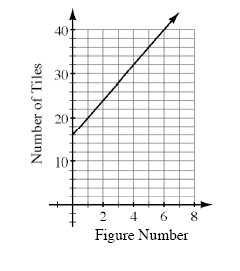The graph at right represents the number of tiles in a tile pattern. Homework Help ✎

1. Based on the information in the graph, how many tiles are being added each time (that is, what is the growth factor of the pattern)? Pay close attention to the scale of the axes.

2. How many tiles are in Figure 0?

3. How would the line change if the pattern grew by 12 tiles each time instead?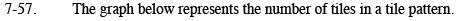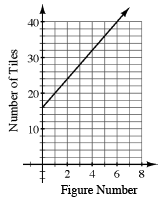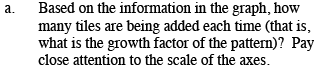How many tiles are in Figures 1 and 2? What is the difference?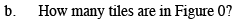Since x represents the figure number, find y when x = 0.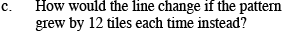If Figure 0 has 16 tiles, how many tiles would Figures 2 and 3 have?
Where would these points be on the graph?